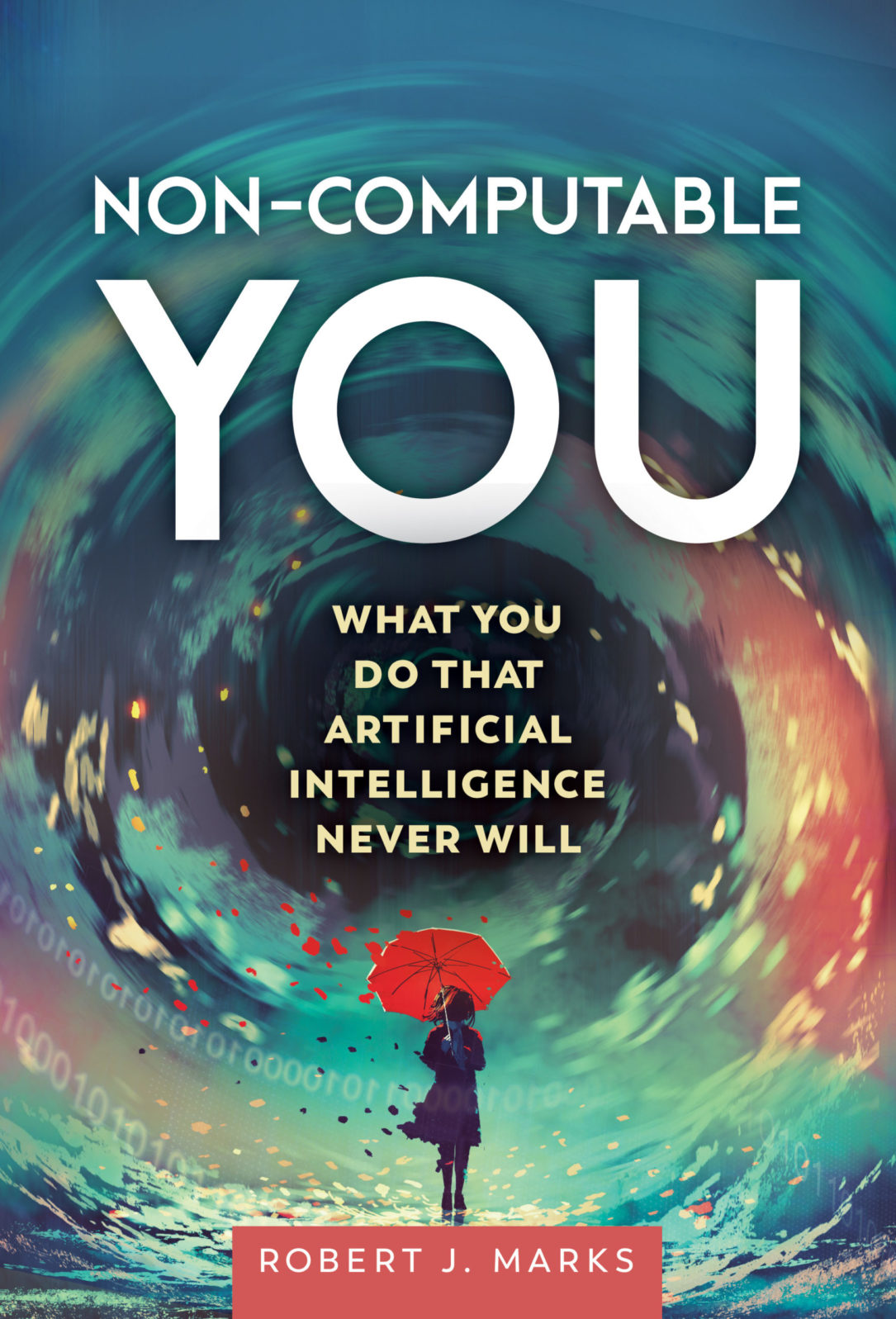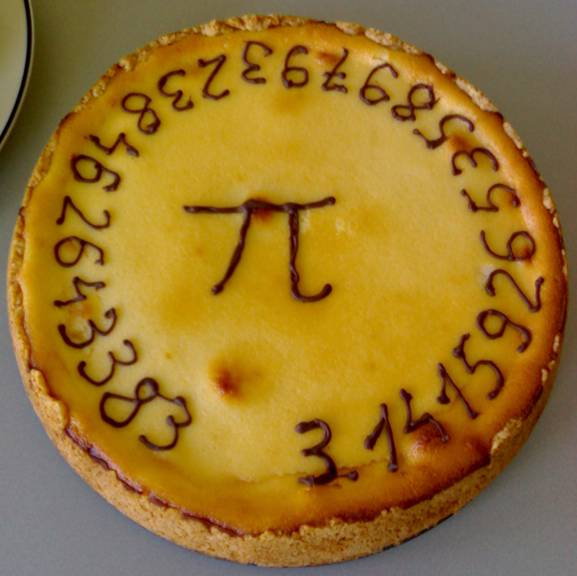Mind Matters Natural and Artificial Intelligence News and Analysis

# 4. How Almost Any Number Can Encode the Library of Congress

That’s a weird, counterintuitive — but quite real — consequence of the concept of infinity in math

We are used to dealing with simple numbers, like ½ and 2. Most numbers are not that simple.

Most numbers, like 0.847859028378490… go on forever and ever without repeating or showing any pattern. Note that such numbers, called irrational numbers, have an infinite number of digits. And there are a lot of them. The number 0.847859028378490… for example differs from the number 0.847859023378490… (See if you can spot the difference.) If two numbers differ only at the billionth decimal and are otherwise the same, they are different numbers.(Non-Computable You (Discovery Institute Press, 2022) by Robert J. Marks is available here.)

Because an irrational number is infinitely long — and we have seen in the first three posts that weird things happen with infinity — we’d expect something weird to happen with irrational numbers. We’ll now show that the Library of Congress is digitally represented on almost every irrational number. That’s weird.

Numbers can be written in base 10 or base 2. Base 2 numbers have only ones and zeros as digits. Binary representation serves us best in the illustration to follow. How to transform from one base to another is not important. It’s sufficient to know that it can be done. For example, the binary number 0.1010 transforms in base 10 to 0.6250.

Let’s generate a random number between zero and one by flipping a fair coin. If the coin turns up heads, that’s a one. A tails is zero. The coin is flipped 3 times and the results are heads, tails, heads. According to our rules, this will correspond to the binary decimal 0.101. Three more flips gives all tails. Thus three zeros are added to the end and we get 0.101000. The coin is then flipped forever and a real number (between zero and one) is randomly generated.

Now we get to ask some interesting questions. Will 50 ones in a row be generated eventually by coin flipping? It might take a long time, but the answer is obviously yes. How about a million ones in a row? Don’t forget, the coin flipping continues forever. So yes. Eventually. we get a million ones in a row.

To generate the random number, the fair coin must be flipped an infinite number of times. Most assuredly, this number is said to be irrational. It contains no repetitions or patterns.

All documents in English can be reduced to a sequence of ones and zeros. ASCII (American Standard Code for Information Interchange) uses eight bits for each letter, character and punctuation mark in the English language. For example, the letter B is assigned the eight bit number 01000010. The name “Bob” in ASCII is 010000100110111101100010. In generating bits for the irrational number by coin flipping, will we eventually reproduce this ASCII code for “Bob”? It might take some time, but most assuredly the answer is yes. What about the ASCII version of this entire article? Eventually yes. The entire book Moby Dick? The King James version of the Bible? Yes and yes. The entire contents of the Library of Congress?

Yes.

Almost every number between zero and one randomly chosen by coin flipping will at some point contain the binary encoding of the Library of Congress. A math nerd would say that this is assured with probability one.

What, though, about numbers like ½ =0.5? It doesn’t contain the Library of Congress. But randomly choosing 0.5, equal to 0.100000000000000000… in binary, has a zero chance of occurring. It requires an infinite number of zeros in a row after the first one. That has a zero chance of happening. This is also true of all the rational numbers between zero and one that don’t contain the entire Library of Congress.

The cause of our strange observation is infinity. When infinity is assumed to exist, weird things can happen. Most real numbers require infinite precision to define. And, as discussed, infinity does not exist.

The most famous number that goes on forever without repeating is π = 3.14159… Like all irrational numbers, π is never used to infinite precision. If we ask for π gallons of gas to be added to our car, we mean add 3.14 gallons of gas. In reality, irrational numbers are always rounded.The first digits of π (pi) decorating a pie/Public Domain

Because they require an infinite number of digits to uniquely define, irrational numbers do not exist in reality. Since most real numbers are irrational, we can even make the oxymoronic claim that most real numbers are not real.

Like the model of infinity, irrational numbers only exist as a mathematical model in our minds. The fact that all irrational numbers contain at some point the ASCII representation of the entire Library of Congress is sufficiently weird to rule out the idea that irrational numbers are real. Note that the same number contains somewhere the encoding of the Library of Congress backwards and at other places all the versions of the Library of Congress that are off by one bit. All this in a single number of infinite length.

The consequences of infinity are strange and counterintuitive.

Next: In the last installment of this series, we show that (1) some infinities are bigger than other infinities and (2) there is no biggest infinity.

Here are the earlier instalments in this series:

Part 1: Why infinity does not exist in reality. A few examples will show the absurd results that come from assuming that infinity exists in the world around us as it does in math. In a series of five posts, I explain the difference between what infinity means — and doesn’t mean — as a concept.

Part 2. Infinity illustrates that the universe has a beginning. The logical consequences of a literally infinite past are absurd, as a simple illustration will show. The absurdities that an infinite past time would create, while not a definitive mathematical proof, are solid evidence that our universe had a beginning.

and

Part 3: In infinity, lines and squares have an equal number of points Robert J. Marks: We can demonstrate this fact with simple diagram. This counterintuitive result, driven by Cantor’s theory of infinities is strange. Nevertheless, it is a valid property of the infinite.

You may also wish to read: Yes, you can manipulate infinity in math. The hyperreals are bigger (and smaller) than your average number — and better! (Jonathan Bartlett)

1x